# The Area Enclosed by Hamiltonian Cycles on Highly Regular Planar Graphs

This post was inspired by some doodles I drew on a notepad during some meetings at work. I vividly remember watching the youtube video which introduced me to the mathematics of juggling and listening to the lecturer spend several minutes justifying why juggling ought to have a mathematics. I often think back to this when observing phenomena in my day to day life. I think it is valuable to look at simple patterns around us and consider them from a mathematical perspective - even if we skimp on the formality and rigor.

# The Doodles

During a meeting, I doodled some square-ish grids of dots on my notepad. I then started connecting these dots with vertical and horizontal lines. As I kept connecting, I ended with a Hamiltonian cycle over the dots. For those who haven’t taken any graph theory, a Hamiltonian cycle is a cycle (a path which starts and ends in the same place) which visits every vertex in a graph exactly once.

The cycle I’d drawn was quite irregular so I redrew the same grid of dots and tried to find a more symmetric way to connect them. Here are a three examples of such cycles on a six by six grid of dots.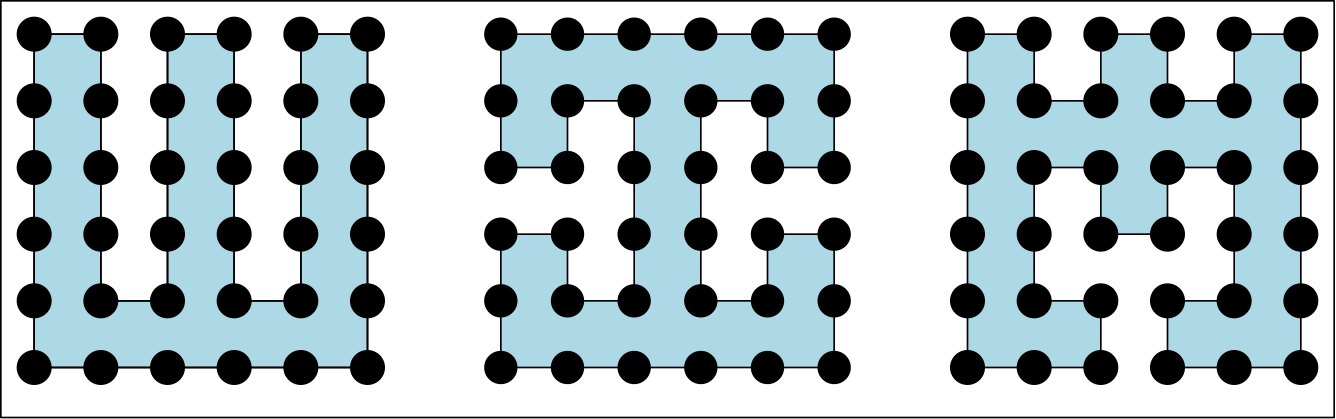# An Unexpected Pattern

As I looked at these grids, I noticed something surprising - they all enclosed the same number of cells. If we take the edge length to 1 then each one encloses 17 squares of area.

I started experimenting with larger grids found that for a given set of vertices, the area enclosed by a Hamiltonian cycle never seemed to depend on my choice of cycle.

# “Proof”

I mentioned this to a coworker who suggested a useful paradigm shift. Instead of thinking about the dots in isolation, think about them as the centers of squares whose side length matches the edge length in our grid. Now, at each vertex, the angle of the line going through it determines how much of its square is enclosed by the cycle. For convenience, of notation I’m going to assume that the cycle starts in the bottom left corner of the graph and goes up. If you prefer to go the other way, just swap some negative signs and the math is all the same.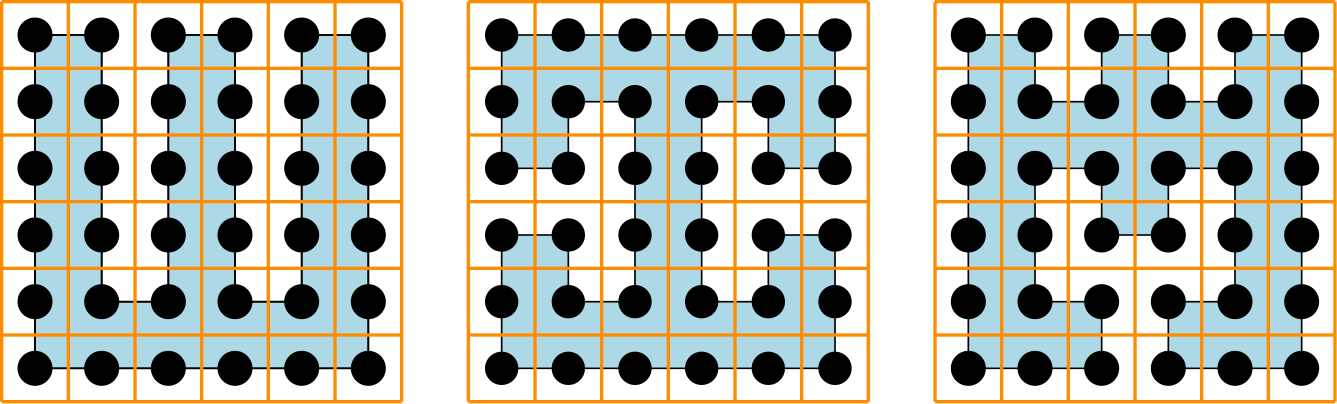When the line is straight (0°), half the cell containing that dot is enclosed by the cycle. When the line through a vertex turns to to the right (90°), one quarter of the cell is enclosed by the cycle. Finally, when the line through a vertex turns left, (-90°), three quarters of the cell is enclosed by the cycle.

More concretely, we can express the area enclosed by a vertex of angle $\theta$

$\frac{1}{2} - \frac{1}{4} * \frac{\theta}{90}$

This means we can compute the area enclosed by the cycle as

$\sum_{\text{vertices}} \left(\frac{1}{2} - \frac{1}{4} * \frac{\theta}{90}\right)$

We can do a little rearrangement to express this sum as

$\sum_{\text{vertices}} \left(\frac{1}{2}\right) - \frac{1}{360} * \sum_{\text{vertices}} \left( \theta \right)$

This is where we get to bring in a fun fact. In a closed loop whose corners are a square and which does not cross itself, the sum of the angles is 360°. This means that everything in the above expression is a constant which means the area enclosed by the curve is a constant.

# Extensions

This “proof” applies equally well if we replace our grid graph with a tiling of equaliteral triangles.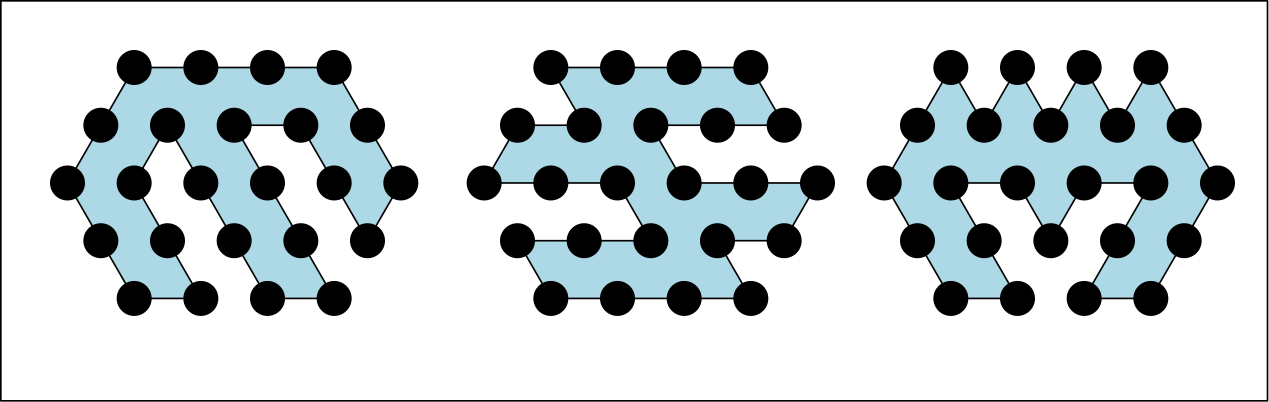A few of the constants change but the overall structure of looking at the area bounded by a angle through each vertex remains the same.

Both of the examples I’ve shown in this post have a certain regularity which makes this argument flow very naturally. I’m curioius if it is possible to characterize the this regularity and describe other families of graph which tesselate the plane and for which the area enclosed by all hamilton cycles on a subgraph is constant.

I have a hypothesis that the condition required on the original graph is “the graph is planar and all cells in the original graph are the same”. I use cells here to mean cycles which cannot be subdivided into unions of (geometrically) smaller cycles. This hypothesis feel intuitive to me and covers cases like affine transformations of known tesselations.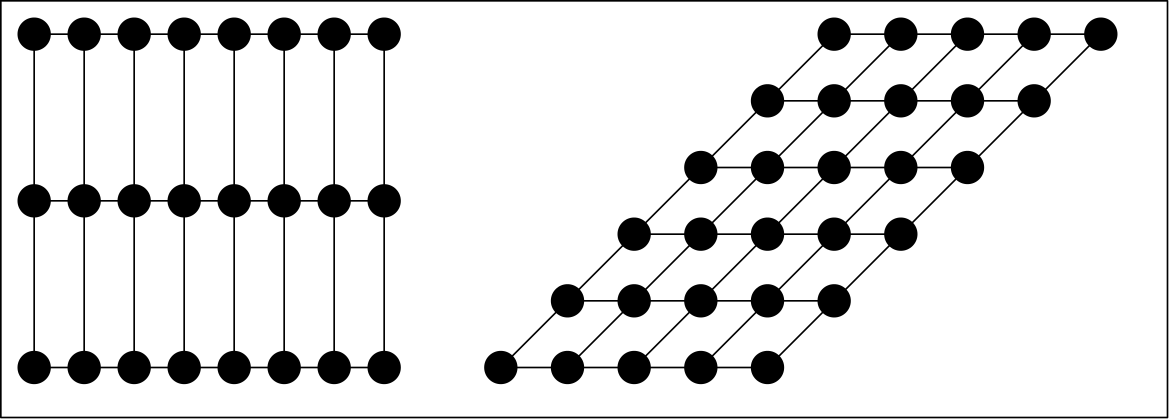I would not be suprised if an even looser restriction is sufficient like “all cells have the same number of edges and bound the same area.” I need to think more about what such a tesselation looks like.

# “Holes” in the Subgraph

My math knowledge is getting thin here so this subsection will probably use some terms incorrectly.

All of the graphs I’ve shown in this post have had no gaps in their vertices. That is to say, if they can be generated by tiling of the cartesian plane with one of the tesselations discussed, drawing a close loop, and taking the subgraph whose vertices are enclosed in the loop. But what about subgraphs like the one below: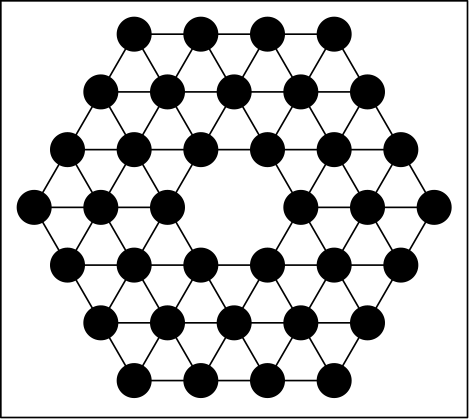Unfortunately, the property does not hold on all such graphs. It is possible for the missing vertex to be inside or outside the shape enclosed by the Hamiltonian Cycle. These two scenarios lead to different amounts of enclosed space. In the following example, the cycle on the left encloses 34 triangles and the cycle on the right encloses 36 triangles.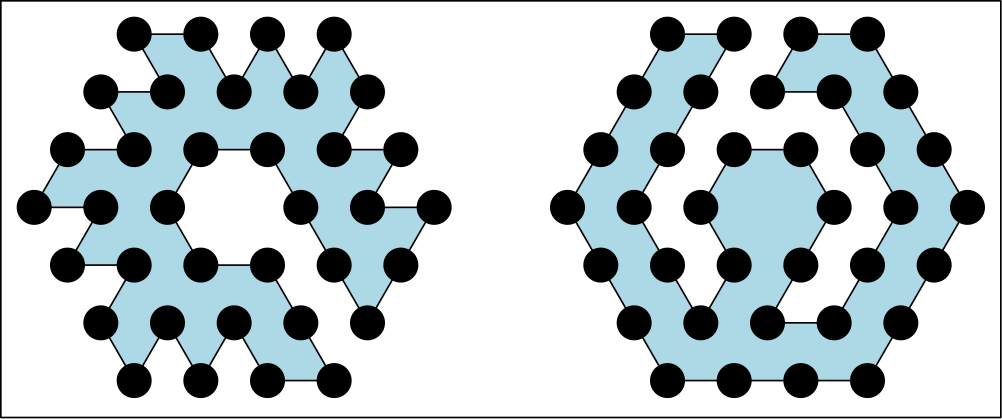# How Much is Enclosed

In the case of a square grid which is n verticies wide and m verticies high, the cycle which encloses the maximum area is the perimiter with $(n-1) * (m - 1)$. The Hamiltonian cycle on such grids falls short of this maximum by half the number of non-perimeter vertices. This value is always an integer since a square grid where both dimensions are odd has no Hamiltonian cycle.

In the case of a regular tiling of equalerateral triangles, the the Hamiltonian Cycle falls short of the perimeter by as many triangular cells as there are non-perimeter vertices.

I suspect that in a tiling of n-gons, the number of cells that the Hamaltonian cycle will fall short of the perimeter will be $\frac{\text{number of interanl vertices}}{n-2}$ . My hand-wavey explanation of this guess is that it takes n-2 added vertices to carve out a cell from the perimeter. If this is true, it would have interesting implications on determining whether graphs of certain families have Hamiltonian cycles or not simply by counting the internal vertices

Written on June 17, 2017